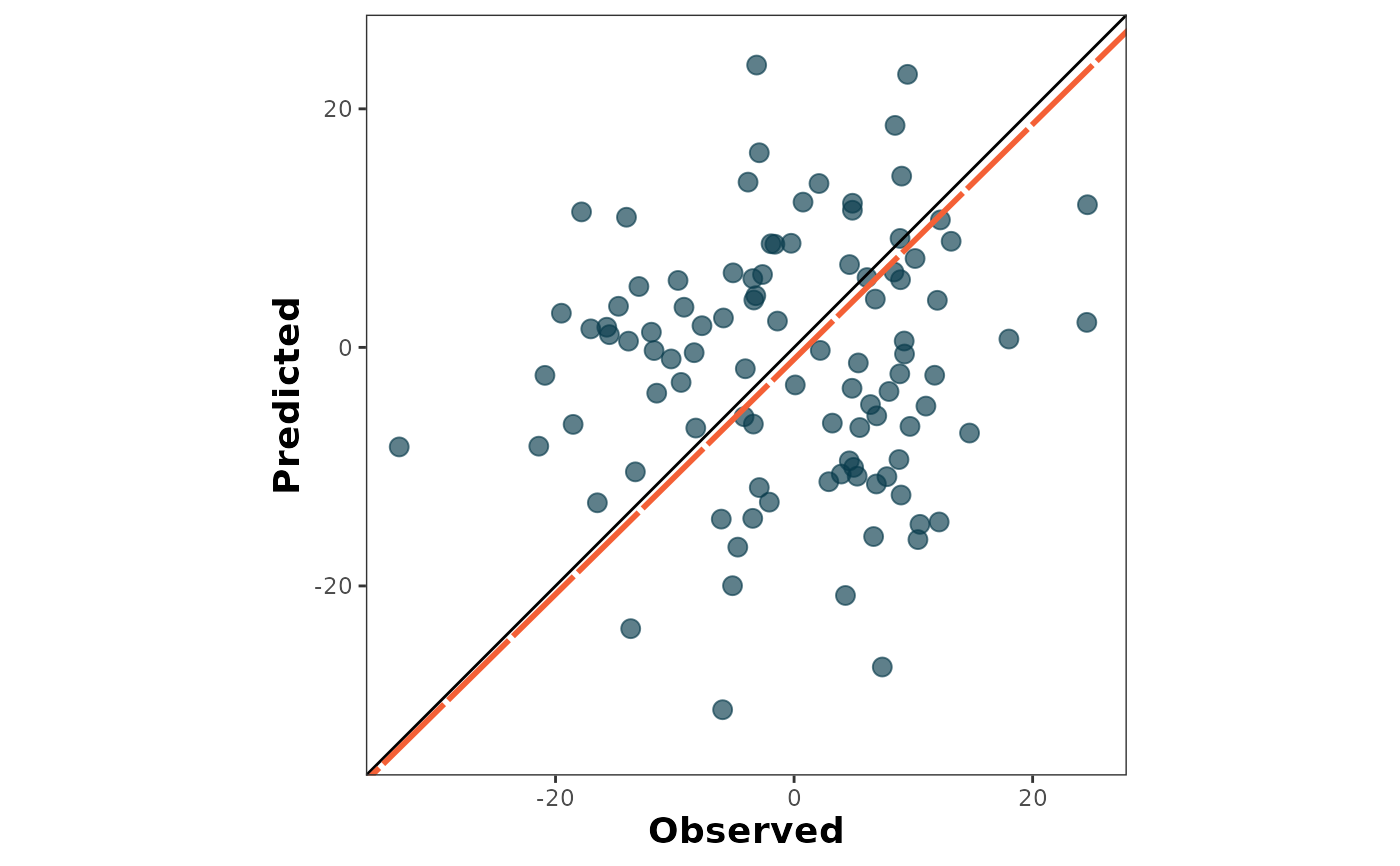It draws a scatter plot of predictions and observations with alternative axis orientation (P vs. O; O vs. P).

## Usage

scatter_plot(
data = NULL,
obs,
pred,
orientation = "PO",
print_metrics = FALSE,
metrics_list = NULL,
position_metrics = c(x = NULL, y = NULL),
print_eq = TRUE,
position_eq = c(x = NULL, y = NULL),
eq_color = NULL,
shape_type = NULL,
shape_size = NULL,
shape_color = NULL,
regline_type = NULL,
regline_size = NULL,
regline_color = NULL,
na.rm = TRUE
)

## Arguments

data

(Optional) argument to call an existing data frame with the data.

obs

Vector with observed values (numeric).

pred

Vector with predicted values (numeric).

orientation

Argument of class string specifying the axis orientation, PO for predicted vs observed, and OP for observed vs predicted. Default is orientation = "PO".

print_metrics

boolean TRUE/FALSE to embed metrics in the plot. Default is FALSE.

metrics_list

vector or list of selected metrics to print on the plot.

position_metrics

vector or list with '(x,y)' coordinates to locate the metrics_table into the plot. Default : c(x = min(obs), y = 1.05*max(pred)).

print_eq

boolean TRUE/FALSE to embed metrics in the plot. Default is FALSE.

position_eq

vector or list with '(x,y)' coordinates to locate the SMA equation into the plot. Default : c(x = 0.70 max(x), y = 1.25*min(y)).

eq_color

string indicating the color of the SMA-regression text.

shape_type

integer indicating the shape type for the data points.

shape_size

number indicating the shape size for the data points.

shape_color

string indicating the shape color for the data points.

regline_type

string or integer indicating the SMA-regression line-type.

regline_size

number indicating the SMA-regression line size.

regline_color

string indicating the SMA-regression line color.

na.rm

Logic argument to remove rows with missing values (NA). Default is na.rm = TRUE.

## Value

an object of class ggplot.

## Details

It creates a scatter plot of predicted vs. observed values. The plot also includes the 1:1 line (solid line) and the linear regression line (dashed line). By default, it places the observed on the x-axis and the predicted on the y-axis (orientation = "PO"). This can be inverted by changing the argument orientation = “OP". For more details, see online-documentation

ggplot,geom_point,aes
# \donttest{